# Electronics and Communication Engineering - Networks Analysis and Synthesis

1.

A dielectric does not have leakage current.

 A. True B. False

Explanation:

All dielectrics have some leakage current.

2.

A series RLC circuit has a resonant frequency of 1000 Hz. The maximum voltage across C is likely to occur at a frequency of about

 A. 1000 Hz B. 2000 Hz C. 1025 Hz D. 975 Hz

Explanation:

Maximum voltage across capacitance occurs at a frequency slightly less than resonant frequency.

3.

The resistance of a 230 V, 100 W lamp is

 A. 529 Ω B. 2300 Ω C. 5290 Ω D. 23 Ω

Explanation: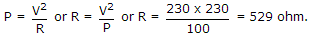4.

System function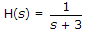, For a signal sin 2t, the steady state response is

 A. 1/8 B. ∞ C. 0 D. 8

Explanation: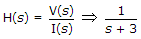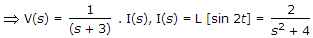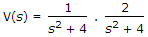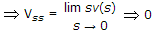.

5.

A network has two branches in parallel. One branch contains impedance Z1 and the other branch has impedance Z2. If it is fed from an ac voltage V of frequency f, the current through Z1 depends, on

 A. V, Z1, Z2 B. V, Z1 C. Z1, Z2 D. V, Z1, f